Home

### Sequences and sets (11Bxx)

• Article
• #####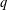$q$ -DEFORMED RATIONALS AND$q$ -CONTINUED FRACTIONS
• Forum of Mathematics, Sigma, Volume 8

### Diophantine equations (11Dxx)

• Article
• ##### TRANSFORMATION FORMULAS FOR THE NUMBER OF REPRESENTATIONS OF$n$ BY LINEAR COMBINATIONS OF FOUR TRIANGULAR NUMBERS
• Bulletin of the Australian Mathematical Society, Volume 102, Issue 1

• Article
• ##### Equidistribution of Farey sequences on horospheres in covers of$\text{SL}(n+1,\mathbb{Z})\backslash \text{SL}(n+1,\mathbb{R})$ and applications
• Ergodic Theory and Dynamical Systems, First View

### Forms and linear algebraic groups (11Exx)

• Article
• ##### TRANSFORMATION FORMULAS FOR THE NUMBER OF REPRESENTATIONS OF$n$ BY LINEAR COMBINATIONS OF FOUR TRIANGULAR NUMBERS
• Bulletin of the Australian Mathematical Society, Volume 102, Issue 1

• Article
• ##### PRIME-UNIVERSAL QUADRATIC FORMS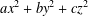$ax^{2}+by^{2}+cz^{2}$ AND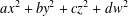$ax^{2}+by^{2}+cz^{2}+dw^{2}$
• Bulletin of the Australian Mathematical Society, Volume 101, Issue 1

### Discontinuous groups and automorphic forms (11Fxx)

• Article
• ##### ANALYTIC PROPERTIES OF EISENSTEIN SERIES AND STANDARD$L$ -FUNCTIONS
• Nagoya Mathematical Journal, First View

• Article
• ##### An exceptional Siegel–Weil formula and poles of the Spin L-function of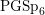$\text{PGSp}_{6}$
• Compositio Mathematica, Volume 156, Issue 6

### Probabilistic theory: distribution modulo $1$; metric theory of algorithms (11Kxx)

• Article
• ##### Equidistribution of Farey sequences on horospheres in covers of$\text{SL}(n+1,\mathbb{Z})\backslash \text{SL}(n+1,\mathbb{R})$ and applications
• Ergodic Theory and Dynamical Systems, First View

### Exponential sums and character sums (11Lxx)

• Article
• ##### One-Level Density of Low-lying Zeros of Quadratic and Quartic Hecke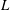$L$ -functions
• Canadian Journal of Mathematics, Volume 72, Issue 2

### Zeta and $L$-functions: analytic theory (11Mxx)

• Article
• ##### ANALYTIC PROPERTIES OF EISENSTEIN SERIES AND STANDARD$L$ -FUNCTIONS
• Nagoya Mathematical Journal, First View

• Article
• ##### Ideal Uniform Polyhedra in$\mathbb{H}^{n}$ and Covolumes of Higher Dimensional Modular Groups
• Canadian Journal of Mathematics, First View

### Algebraic number theory: global fields (11Rxx)

• Article
• #####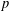$p$ -ADIC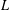$L$ -FUNCTIONS FOR UNITARY GROUPS
• Forum of Mathematics, Pi, Volume 8

### Algebraic number theory: local and $p$-adic fields (11Sxx)

• Article
• ##### CHARACTERIZING THE MOD-$\ell$ LOCAL LANGLANDS CORRESPONDENCE BY NILPOTENT GAMMA FACTORS
• Nagoya Mathematical Journal, First View

• Article
• ##### Attractors associated to a family of hyperbolic$p$ -adic plane automorphisms
• Ergodic Theory and Dynamical Systems, First View

• Article
• ##### WAVE FRONT HOLONOMICITY OF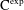$\text{C}^{\text{exp}}$ -CLASS DISTRIBUTIONS ON NON-ARCHIMEDEAN LOCAL FIELDS
• Forum of Mathematics, Sigma, Volume 8

### Connections with logic (11Uxx)

• Article
• ##### WAVE FRONT HOLONOMICITY OF$\text{C}^{\text{exp}}$ -CLASS DISTRIBUTIONS ON NON-ARCHIMEDEAN LOCAL FIELDS
• Forum of Mathematics, Sigma, Volume 8